## DH89. EGGP to EGCB Fuel Problem

Check here to keep abreast of FSAirlines updates.

Moderators: The Ministry, Guru's

simondix
Concorde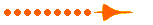Posts: 1826
Joined: 10 Aug 2007, 08:54
Location: Redditch, Worcestershire, UK

### DH89. EGGP to EGCB Fuel Problem

I have twice tried to fly the above flight. I have loaded first time 56 kg then second time 72 kg. The client has accepted this but when flying I run out of fuel after about two minutes.
Simon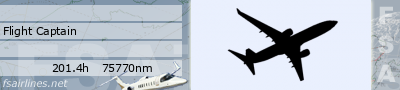'The trouble with the speed of light is it gets here too early in the morning!' Alfred. E. Neuman

Effoh
ConcordePosts: 745
Joined: 28 Oct 2005, 07:13
Location: Troon, Ayrshire

### Re: DH89. EGGP to EGCB Fuel Problem

Hi Simon,

Is this just with FSAirlines or does it happen offline as well?

Rgds,

Colin

DaveB
The Ministry
Posts: 30457
Joined: 17 Jun 2004, 20:46
Location: Pelsall, West Mids, UK
Contact:

### Re: DH89. EGGP to EGCB Fuel Problem

Hi Simon..

Not sure what's going on with AERN. It has 56kg FOB from your last flight in Sep.. plenty to get you to Barton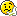Has your system changed since last Sep?? TBH.. I've absolutely no idea why it's running out of fuel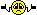Can you check with the fuel gauge to make sure it's taken fuel. I'm off back to Norwich in a min or so and have to pull my finger out or I'll run into 'work' time.

Nice hammer Colin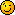ATB
DaveB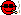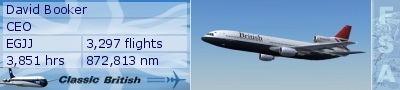Old sailors never die.. they just smell that way!

Effoh
ConcordePosts: 745
Joined: 28 Oct 2005, 07:13
Location: Troon, Ayrshire

### Re: DH89. EGGP to EGCB Fuel Problem

DaveB wrote:Nice hammer ColinMy Grandad thought so too mate!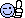Simon,

Have just loaded 56kgs in Dave G's FSX Rapide and it does the flight you're trying to do fine offline. Guess it's the clientC

simondix
ConcordePosts: 1826
Joined: 10 Aug 2007, 08:54
Location: Redditch, Worcestershire, UK

### Re: DH89. EGGP to EGCB Fuel Problem

Very Odd. As you may know I have multiple FS9 setups. Only my DH89v2 was affected in my Silverwings set up. I flew the flight using the DH89 v1. No problem. Yes the V2 was the same offline. I then tried the next flight EGCB to EGNM. Same problem. Took the DH89v2 config out of my Goldenwings, no change. Flew the flight in my Goldenwings set up in the V2. No problem.

Simon'The trouble with the speed of light is it gets here too early in the morning!' Alfred. E. Neuman

DaveB
The Ministry
Posts: 30457
Joined: 17 Jun 2004, 20:46
Location: Pelsall, West Mids, UK
Contact:

### Re: DH89. EGGP to EGCB Fuel Problem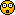Come again??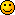Wish I could offer some advice mate but I've no idea how the client handles multiple installs. It should see only the active but I couldn't be sure. Certainly, it's not the v2 Rapide.. this is the only one I use and have ever used. An odd one and no mistakin'!ATB
DaveBOld sailors never die.. they just smell that way!

simondix
ConcordePosts: 1826
Joined: 10 Aug 2007, 08:54
Location: Redditch, Worcestershire, UK

### Re: DH89. EGGP to EGCB Fuel Problem

I presume it is the client. I ave flown the Rapide with this set up all the time. See what happens next time.
Simon'The trouble with the speed of light is it gets here too early in the morning!' Alfred. E. Neuman

DaveB
The Ministry
Posts: 30457
Joined: 17 Jun 2004, 20:46
Location: Pelsall, West Mids, UK
Contact:

### Re: DH89. EGGP to EGCB Fuel Problem

Ok mateATB
DaveBOld sailors never die.. they just smell that way!

simondix
ConcordePosts: 1826
Joined: 10 Aug 2007, 08:54
Location: Redditch, Worcestershire, UK

### Re: DH89. EGGP to EGCB Fuel Problem

Right. Tried again in my Silverwings set up and same problem occurred. However in the older Rapide download it did work. I am going to paste the cfgs here and see if anybody can see a problem. The first one is my cfg that does not work. It does in Goldenwings.

No.1

[General]
performance=Length: 82 feet\nWing span: 115ft\nWeight: max, 54,983lbs\nPower: Bristol Centaurus\nDetails: 34\/seater freight combi\n
editable=1
category=airplane

[pitot_static]
vertical_speed_time_constant = 2

[exits]
number_of_exits=2
exit_rate.0=0.1
exit_rate.1=0.1

[WEIGHT_AND_BALANCE]
reference_datum_position=0.000000,0.000000,0.000000
empty_weight_CG_position= -0.600, 0.000, -4.500
empty_weight=36815.00000
max_gross_weight=54993.0
max_number_of_stations=6
empty_weight_roll_MOI=253496.00000
empty_weight_pitch_MOI=309900.00000
empty_weight_yaw_MOI=451646.00000
empty_weight_coupled_MOI=0.00000
station_load.0= 998, 30.000, 0.000, -4.000, NoseHold
station_load.1= 2, 25.200, 0.000, -2.900, Pilots
station_load.2= 2998, 11.125, 0.000, -4.000, FwdHold
station_load.3= 2145, -0.600, 0.000, -4.000, PaxRows-1-3
station_load.4= 5610, -7.500, 0.000, -4.000, 34-Pax
station_load.5= 1496, -18.670, 0.000, -4.000, AftHold

[flight_tuning]
cruise_lift_scalar=1.00000
parasite_drag_scalar=1.00000
induced_drag_scalar=1.00000
elevator_effectiveness=1.00000
aileron_effectiveness=1.00000
rudder_effectiveness=1.00000
pitch_stability=1.00000
roll_stability=1.00000
yaw_stability=1.00000
elevator_trim_effectiveness=1.00000
aileron_trim_effectiveness=1.00000
rudder_trim_effectiveness=1.00000
p_factor_on_yaw=1.00000
torque_on_roll=1.00000
gyro_precession_on_yaw=1.00000
gyro_precession_on_pitch=1.0
hi_alpha_on_roll=1.00000
hi_alpha_on_yaw=1.00000
gyro_precession_on_roll=1.00000

[fuel]
LeftMain= -0.600, -10.000, -1.300, 600.000, 0.000
RightMain= -0.600, 10.000, -1.300, 600.000, 0.000
fuel_type=1
number_of_tank_selectors=1
electric_pump=0
fuel_dump_rate=0.02000
anemometer_pump=0
engine_driven_pump=0
manual_pump=0

[airplane_geometry]
wing_area=1200.00000
wing_span=115.00000
wing_root_chord=15.79000
wing_dihedral=1.80000
wing_incidence=0.00000
wing_twist=0.00000
oswald_efficiency_factor=0.78600
wing_winglets_flag=0
wing_sweep=1.50000
wing_pos_apex_lon=2.86178
wing_pos_apex_vert=-1.20000
htail_area=180.00000
htail_span=30.20000
htail_pos_lon=-39.03000
htail_pos_vert=-2.00000
htail_incidence=0.00000
htail_sweep=0.00000
vtail_area=166.00000
vtail_span=21.80000
vtail_sweep=0.00000
vtail_pos_lon=-39.77000
vtail_pos_vert=2.50000
elevator_area=66.00000
aileron_area=73.80000
rudder_area=55.00000
elevator_up_limit=20.00000
elevator_down_limit=16.00000
aileron_up_limit=18.00000
aileron_down_limit=14.50000
rudder_limit=23.49000
elevator_trim_limit=19.48000
spoiler_limit=0.00000
spoilerons_available=0
aileron_to_spoileron_gain=0.00000
min_ailerons_for_spoilerons=0.00000
min_flaps_for_spoilerons=0.00000
spoiler_handle_available=0
auto_spoiler_available=0
positive_g_limit_flaps_up=3.10000
negative_g_limit_flaps_up=-2.48000
positive_g_limit_flaps_down=2.17000
negative_g_limit_flaps_down=-1.86000
spoiler_extension_time=1.00000

[contact_points]
static_pitch= 0.000
static_cg_height= 12.000
gear_system_type=1
max_number_of_points=7
tailwheel_lock=0
point.0= 1, 24.500, 0.000, -12.000, 1575, 0, 0.860, 40.300, 0.272, 2.500, 0.679, 5.000, 5.000, 0, 170.0, 175.0
point.1= 1, -1.767, -14.000, -12.000, 1575, 1, 0.860, 0.000, 0.272, 2.500, 0.679, 5.000, 7.000, 2, 170.0, 175.0
point.2= 1, -1.767, 14.000, -12.000, 1575, 2, 0.860, 0.000, 0.272, 2.500, 0.679, 5.000, 7.000, 3
point.3= 2, 0.000, -57.500, 0.000, 1575, 0, 0.000, 0.000, 0.000, 0.000, 0.000, 0.000, 0.000, 5
point.4= 2, 0.000, 57.500, 0.000, 1575, 0, 0.000, 0.000, 0.000, 0.000, 0.000, 0.000, 0.000, 6
point.5= 2, -31.833, 0.000, -10.000, 1575, 0, 0.000, 0.000, 0.000, 0.000, 0.000, 0.000, 0.000, 9
point.6= 2, 34.000, 0.000, -5.333, 1575, 0, 0.000, 0.000, 0.000, 0.000, 0.000, 0.000, 0.000, 4

[LIGHTS]
//Types: 1=beacon, 2=strobe, 3=navigation, 4=cockpit, 10=cabin
light.0=3, 1.500000, -56.500000, -0.400000, fx_navred
light.1=3, 1.500000, 56.500000, -0.400000, fx_navgre
light.2=3, -49.400000, 0.000000, -1.330000, fx_navwhi
light.3=2, -23.300000, 0.000000, -0.700000, fx_beacon
light.4=2, 12.800000, 0.000000, -10.500000, fx_beacon
light.5=4, 24.000000, 0.000000, -3.300000, fx_vclight_as57-c
light.6=10, 5.300000, 0.000000, -4.000000, fx_vclight_as57-2
light.7=10, -17.900000, 0.000000, -4.000000, fx_vclight_as57-2
light.8=10, 18.500000, 0.000000, -4.000000, fx_vclight_as57-1
light.9=10, -29.100000, 0.000000, -4.000000, fx_vclight_as57-1

[EFFECTS]
startup=fx_engstrt_as57
contrail=fx_A_I_Contrail

[Flaps.0]
type= 1
system_type=1
span-outboard= 0.500
extending-time= 5.0
damaging-speed=180.0
blowout-speed=225.0
flaps-position.0=0.00
flaps-position.1=13.33, 153.0
flaps-position.2=26.67, 140.3
flaps-position.3=40.00, 130.0
lift_scalar=1.00
drag_scalar=1.00
pitch_scalar=1.00

[GeneralEngineData]
engine_type=0
Engine.0=10.300, -14.000, -1.400
Engine.1=10.300, 14.000, -1.400
fuel_flow_scalar=0.78200
min_throttle_limit=0.00000

[piston_engine]
power_scalar =1.00000
cylinder_displacement =181.66667
compression_ratio =7.20000
number_of_cylinders =18
max_rated_rpm =2700.00000
max_rated_hp =2625.00000
fuel_metering_type =0
cooling_type =0
normalized_starter_torque =0.03292
turbocharged =1
max_design_mp =57.27000
min_design_mp =8.76000
critical_altitude =9800.00000
emergency_boost_type =0
emergency_boost_mp_offset =0.00000
emergency_boost_gain_offset =0.00000
fuel_air_auto_mixture =1
auto_ignition =0
max_rpm_mechanical_efficiency_scalar =1.00000
idle_rpm_mechanical_efficiency_scalar =1.00000
max_rpm_friction_scalar =1.00000
idle_rpm_friction_scalar =1.00000
emergency_boost_duration =0.00000
BestPowerSpecificFuelConsumption=0.60000
detonation_onset=63.25000

[propeller]
thrust_scalar =1.00000
propeller_type =0
rotation = -1,-1
propeller_diameter =16.00000
propeller_moi =304.65467
beta_max =65.00000
beta_min =18.00000
min_gov_rpm =1400.00000
prop_tc =0.05000
gear_reduction_ratio =2.15000
fixed_pitch_beta =30.00000
low_speed_theory_limit =80.00000
prop_sync_available =0
prop_deice_available =0
prop_feathering_available =1
prop_auto_feathering_available =0
min_rpm_for_feather =700.00000
beta_feather =88.50000
power_absorbed_cf =0.90000
defeathering_accumulators_available =0
prop_reverse_available =0
minimum_on_ground_beta =12.00000
minimum_reverse_beta =0.00000
feathering_switches=1

[electrical]
max_battery_voltage = 24.0
generator_alternator_voltage = 28.0
max_generator_alternator_amps = 60.0
electric_always_available = 0

[Reference Speeds]
flaps_up_stall_speed=89.99996
full_flaps_stall_speed=86.99996
cruise_speed=190.00000
max_indicated_speed=265.00000
max_mach=0.37000

[keyboard_response]
elevator=150.000000,250.000000
aileron=150.000000,250.000000
rudder=150.000000,250.000000

[Views]
eyepoint=26.298720,-1.009262,-2.104314

[autopilot]
autopilot_available=1
flight_director_available=0
default_vertical_speed=700
autothrottle_available=0
pitch_takeoff_ga=8
max_pitch=10
max_pitch_acceleration=1
max_pitch_velocity_lo_alt=2
max_pitch_velocity_hi_alt=1.5
max_pitch_velocity_lo_alt_breakpoint=20000
max_pitch_velocity_hi_alt_breakpoint=28000
max_bank=15
max_bank_acceleration=1.3
max_bank_velocity=2
max_throttle_rate=0.1
nav_proportional_control=9
nav_integrator_control=0.25
nav_derivative_control=0
nav_integrator_boundary=2.5
nav_derivative_boundary=0
gs_proportional_control=9.52
gs_integrator_control=0.26
gs_derivative_control=0
gs_integrator_boundary=0.7
gs_derivative_boundary=0
yaw_damper_gain=0
autothrottle_arming_required=0
autothrottle_takeoff_ga=1
autothrottle_max_rpm=90
default_pitch_mode=0
default_bank_mode=0
use_no_default_bank=1
use_no_default_pitch=1

Audio.1=1
Com.1=1, 0
Com.2=1, 0
Nav.1=1, 0, 1
Nav.2=1, 0, 0
Transponder.1=1
Marker.1=1

[direction_indicators]
direction_indicator.0=2

[brakes]
toe_brakes_scale=1.00000
parking_brake=1
auto_brakes=0
hydraulic_system_scalar=0.00000
differential_braking_scale=0.00000

[gear_warning_system]
gear_warning_available=0
pct_throttle_limit=0.00000
flap_limit_power=14.99717
flap_limit_idle=29.99434

[hydraulic_system]
normal_pressure=2000.00000
electric_pumps = 0
engine_map = 1,1,0,0

[stall_warning]
type=1

[attitude_indicators]
attitude_indicator.0=2

[turn_indicators]
turn_indicator.0=1,0

[forcefeedback]
gear_bump_nose_magnitude=6000
gear_bump_nose_direction=18000
gear_bump_nose_duration=250000
gear_bump_left_magnitude=6000
gear_bump_left_direction=35500
gear_bump_left_duration=250000
gear_bump_right_magnitude=6000
gear_bump_right_direction=500
gear_bump_right_duration=250000
ground_bumps_magnitude1=3250
ground_bumps_angle1=8900
ground_bumps_intercept1=5
ground_bumps_slope1=0.48
ground_bumps_magnitude2=750
ground_bumps_angle2=9100
ground_bumps_intercept2=0.075
ground_bumps_slope2=1
crash_magnitude1=10000
crash_direction1=1000
crash_magnitude2=10000
crash_direction2=9000
crash_period2=75000
crash_duration2=2500000
stick_shaker_magnitude=5000
stick_shaker_direction=0
stick_shaker_period=111111

Now the one that does work.

No.2

[General]
atc_type=DEHAVILLAND
atc_model=
editable = 1
performance = * Weight: 3516 lbs Max 5500 lbs * Power: 200 hp MIL 200 hp WEP * 4.5g Corner speed: 112 mph * Max speed @ SL: 157 mph * Max speed: 157 mph @ 19500 ft * Climb rate: 1000 ft/min @ 67 mph Flight Model By: Dave Garwood DG Designs 6/2/03 05:40 PM Flight Model Workbook V2.82.99

[pitot_static]
vertical_speed_time_constant = 2

[WEIGHT_AND_BALANCE]
max_gross_weight = 5500
empty_weight = 3346
//reference_datum_position = 0.75, 0, 0
reference_datum_position = 0.65, 0, 0
empty_weight_CG_position = 0.7, 0, 0
CG_forward_limit = 0
CG_aft_limit = 1
max_number_of_stations = 10
station_load.0 = 170, 6.32, 0, 0, // Pilot Weight (lbs), longitudinal, lateral, vertical positions from datum (feet)
station_load.1 = 0, 13.5, 1.3, 0, //
station_load.2 = 0, 13.5, -1.3, 0, //
station_load.3 = 0, 10, 1.3, 0, //
station_load.4 = 0, 10, -1.3, 0, //
station_load.5 = 0, -18, 0, 0, //
station_load.6 = 0, -20, 0, 0, //
station_load.7 = 0, -33, 0, 0, //
station_load.8 = 0, 0, 0, 0, //
station_load.9 = 0, 0, 0, 0, //
empty_weight_pitch_MOI = 3867
empty_weight_roll_MOI = 5966
empty_weight_yaw_MOI = 11473
empty_weight_coupled_MOI = 0

[flight_tuning]
cruise_lift_scalar = 1
parasite_drag_scalar = 1
induced_drag_scalar = 1
elevator_effectiveness = 1
aileron_effectiveness = 1
rudder_effectiveness = 1
pitch_stability = 1
roll_stability = 1
yaw_stability = 1
elevator_trim_effectiveness = 1
aileron_trim_effectiveness = 1
rudder_trim_effectiveness = 1

[GeneralEngineData]
engine_type = 0
Engine.0 = 3.23, -6.1, -1.92
Engine.1 = 3.23, 6.1, -1.92
fuel_flow_scalar = 1
min_throttle_limit=0.000000

[piston_engine]
power_scalar = 1
cylinder_displacement = 93.4333333333333
compression_ratio = 5.25
number_of_cylinders = 6
max_rated_rpm = 2350
max_rated_hp = 200
fuel_metering_type = 1
cooling_type = 0
normalized_starter_torque = 0.303
turbocharged = 0
max_design_mp = 42
min_design_mp = 9
critical_altitude = 19500
emergency_boost_type = 3
emergency_boost_mp_offset = 6.73
emergency_boost_gain_offset = 0.48832730845307
fuel_air_auto_mixture = 0
auto_ignition = 0
max_rpm_mechanical_efficiency_scalar = 1
idle_rpm_mechanical_efficiency_scalar = 1
max_rpm_friction_scalar = 1
idle_rpm_friction_scalar = 1
emergency_boost_duration = 300

[propeller]
thrust_scalar = 1
propeller_type = 1
propeller_diameter = 7.04
propeller_moi = 7.8
beta_max = 40
beta_min = 20
min_gov_rpm = 352.5
prop_tc = 0.01
gear_reduction_ratio = 1
fixed_pitch_beta = 30
low_speed_theory_limit = 77.2442249467081
prop_sync_available = 1
prop_deice_available = 1
prop_feathering_available = 1
prop_auto_feathering_available = 1
min_rpm_for_feather = 700
beta_feather = 88.5
power_absorbed_cf = 0.9
defeathering_accumulators_available = 0
prop_reverse_available = 0
minimum_on_ground_beta = 1
minimum_reverse_beta = -14
rotation = -1, -1

[folding_wings]
wing_fold_system_type = 0
fold_rates = 0.12, 0.11

[TailHook]
tailhook_length = 4
tailhook_position = -15, 0, -1

[contact_points]
max_number_of_points = 9
point.0 = 1, -23.37, 0, -0.334, 1977.953, 0, 0.42, 60, 0.137, 2, 0.7, 0, 0, 0, 0, 0
point.1 = 1, 1.25, -6.08, -6.64, 1968.504, 1, 1.25, 0, 0.41, 2, 0.73, 0, 0, 2, 0, 0
point.2 = 1, 1.25, 6.08, -6.64, 1968.504, 2, 1.25, 0, 0.41, 2, 0.73, 0, 0, 3, 0, 0
point.3 = 2, 10.2066, 0, 0, 2100, 0, 0, 0, 0, 0, 0, 0, 0, 4
point.4 = 2, -22.24, 0, 0.84, 3000, 0, 0, 0, 0, 0, 0, 0, 0, 9
point.5 = 2, -1.135, -24.74, -1.14, 787.402, 0, 0, 0, 0, 0, 0, 0, 0, 5
point.6 = 2, -1.135, 24.74, -1.14, 787.402, 0, 0, 0, 0, 0, 0, 0, 0, 6
point.7 = 2, -1.72, -6.09, -4.9, 787.402, 0, 0, 0, 0, 0, 0, 0, 0, 4
point.8 = 2, -1.72, 6.09, -4.9, 787.402, 0, 0, 0, 0, 0, 0, 0, 0, 4
static_pitch= 15.5049
static_cg_height= 5.59856
tailwheel_lock = 1

[Views]
eyepoint= 6.32, 0, 2.18
[flaps.0] //Trailing Edge Flaps
type = 1
span-outboard = 0.5
extending-time = 5
flaps-position.0 = 0
flaps-position.1 = 15, 80
flaps-position.2 = 30, 77
damaging-speed = 80
blowout-speed = 87
system_type = 1
lift_scalar = 1
drag_scalar = 1
pitch_scalar = 1

[electrical]
max_battery_voltage = 24
generator_alternator_voltage = 28
max_generator_alternator_amps = 160
electric_always_available = 1
flap_motor = 0, 5, 17
gear_motor = 0, 5, 17
autopilot = 0, 5, 17
avionics_bus = 0, 10, 17
avionics = 1, 5, 17
pitot_heat = 0, 2, 17
marker_beacon = 1, 2, 17
gear_warning = 0, 2, 17
fuel_pump = 0, 5, 17
starter1 = 0, 20, 17
starter2 = 0, 20, 17
starter3 = 0, 20, 17
starter4 = 0, 20, 17
light_nav = 0, 5, 17
light_beacon = 0, 5, 17
light_landing = 0, 5, 17
light_taxi = 0, 5, 17
light_strobe = 0, 5, 17
light_panel = 0, 5, 17
prop_sync = 0, 15, 17
auto_feather = 0, 15, 17
auto_brakes = 0, 15, 17
standby_vacuum = 0, 15, 17
hydraulic_pump = 0, 2, 17
fuel_transfer_pump = 0, 5, 17
propeller_deice = 0, 5, 17
light_recognition = 0, 5, 17
light_wing = 0, 5, 17
light_logo = 0, 5, 17

[LIGHTS]
light.0 = 3, -0.242, -22.7788, 4.058, fx_navred
light.1 = 3, -0.242, 22.7788, 4.058, fx_navgre
//light.2 = 3, 9.35, 0, 0.101, fx_navwhi
light.3 = 2, 1.575, -24, 0,
light.4 = 2, 1.575, 24, 0,
light.5 = 4, -3, 0, 2.18,fx_vclight
light.6 = 4, 0, 0, 2.18,fx_vclight
light.7 = 4, 6.32, 0, 2.18,fx_vclight

[keyboard_response]
elevator = 350, 500
aileron = 450, 1000
rudder = 200, 1000

[direction_indicators]
direction_indicator.0 = 1, 0

[exits]
number_of_exits = 1
exit_rate.0 = 0.4

[EFFECTS]
wake = fx_wake
water = fx_spray
dirt = fx_tchdrt
concrete = fx_sparks
touchdown = fx_tchdwn, 1

[autopilot]
default_bank_mode=0
default_pitch_mode=0
autopilot_available = 1
flight_director_available = 0
default_vertical_speed = 1000
autothrottle_available = 0
autothrottle_arming_required = 0
autothrottle_max_rpm = 90
autothrottle_takeoff_ga = 1
pitch_takeoff_ga = 8
max_pitch=10.000000
max_pitch_acceleration=1.000000
max_pitch_velocity_lo_alt=2.000000
max_pitch_velocity_hi_alt=1.500000
max_pitch_velocity_lo_alt_breakpoint=20000.000000
max_pitch_velocity_hi_alt_breakpoint=28000.000000
max_bank=25.000000
max_bank_acceleration=1.800000
max_bank_velocity=3.000000
max_throttle_rate=0.100000
nav_proportional_control=9.000000
nav_integrator_control=0.250000
nav_derivative_control=0.000000
nav_integrator_boundary=2.500000
nav_derivative_boundary=0.000000
gs_proportional_control=9.520000
gs_integrator_control=0.260000
gs_derivative_control=0.000000
gs_integrator_boundary=0.700000
gs_derivative_boundary=0.000000
yaw_damper_gain=0.000000

[fuel]
LeftMain = 0, -3.33, 0, 38, 0
RightMain = 0, 3.33, 0, 38, 0
fuel_type = 1
number_of_tank_selectors = 1
electric_pump=1

[airplane_geometry]
wing_area = 336
wing_span = 48
wing_root_chord = 3.5
wing_dihedral = 3
wing_incidence = 2
wing_twist = -1
oswald_efficiency_factor = 0.947494393860536
wing_winglets_flag = 0
wing_sweep = 0
wing_pos_apex_lon = 1.575
wing_pos_apex_vert = 0
htail_area = 28.4
htail_span = 13.4
htail_pos_lon = -22.11
htail_pos_vert = 0
htail_incidence = 0
htail_sweep = 0
vtail_area = 10.1
vtail_span = 16.84
vtail_sweep = 0
vtail_pos_lon = -22.34
vtail_pos_vert = 0
elevator_area = 20.85
aileron_area = 32.4
rudder_area = 14.9
elevator_up_limit = 30
elevator_down_limit = 20
aileron_up_limit = 20
aileron_down_limit = 15
rudder_limit = 21
elevator_trim_limit = 18
spoiler_limit = 1
spoilerons_available = 0
aileron_to_spoileron_gain = 0
min_ailerons_for_spoilerons = 0
min_flaps_for_spoilerons = 0

[Reference Speeds]
flaps_up_stall_speed = 46.1
full_flaps_stall_speed = 37.4
cruise_speed = 115
max_indicated_speed=310.632813

[forcefeedback]
gear_bump_nose_magnitude = 6000
gear_bump_nose_direction = 18000
gear_bump_nose_duration = 250000
gear_bump_left_magnitude = 6000
gear_bump_left_direction = 35500
gear_bump_left_duration = 250000
gear_bump_right_magnitude = 6000
gear_bump_right_direction = 500
gear_bump_right_duration = 250000
ground_bumps_magnitude1 = 3250
ground_bumps_angle1 = 8900
ground_bumps_intercept1 = 5
ground_bumps_slope1 = 0.48
ground_bumps_magnitude2 = 750
ground_bumps_angle2 = 9100
ground_bumps_intercept2 = 0.075
ground_bumps_slope2 = 1
crash_magnitude1 = 10000
crash_direction1 = 1000
crash_magnitude2 = 10000
crash_direction2 = 9000
crash_period2 = 75000
crash_duration2 = 2500000

[brakes]
toe_brakes_scale=0.640889
parking_brake=1

[hydraulic_system]
electric_pumps=0
engine_map=1,1,0,0
normal_pressure=6.930556

[stall_warning]
type=1

[attitude_indicators]
attitude_indicator.0=1

[turn_indicators]
turn_indicator.0=1,0

Hope this helps
Simon'The trouble with the speed of light is it gets here too early in the morning!' Alfred. E. Neuman

DaveB
The Ministry
Posts: 30457
Joined: 17 Jun 2004, 20:46
Location: Pelsall, West Mids, UK
Contact:

### Re: DH89. EGGP to EGCB Fuel Problem

Hi Simon..

I'm not sure what's going down with the first cfg you posted. Look again at this line and you'll see what I mean..

[General]
performance=Length: 82 feet\nWing span: 115ft\nWeight: max, 54,983lbs\nPower: Bristol Centaurus\nDetails: 34\/seater freight combi\n
editable=1
category=airplane

Length 82ft?
Wingspan 115ft??
Max weight 54,983lbs???
Power Bristol Centaurus????
Details: 34seather freight combi?????

It goes without saying that none of the above equates to the RapideBelow is the correct cfg for the v2 model..

[General]
atc_type=DEHAVILLAND
atc_model=DH89
editable=1
performance=Max speed: 157 mph\nNormal cruising speed: 100-132 mph\nInitial climb: 867 fpm\nService ceiling: 19,500 ft\nRange: 578 nms\n

[pitot_static]
vertical_speed_time_constant=2

[WEIGHT_AND_BALANCE]
max_gross_weight=5500
empty_weight=2934 //3276

reference_datum_position = 0.65, 0, 0
empty_weight_CG_position = 0.7, 0, 0
CG_forward_limit = 0
CG_aft_limit = 1

max_number_of_stations=10
station_load.0="170, 5.8, 0, 0, Pilot"// Pilot Weight (lbs), longitudinal, lateral, vertical positions from datum (feet)
station_load.1="170, 3.2, 1.3, 0, Pax Row1"//
station_load.2="170, 2.2, -1.3, 0, Pax Row 1"//
station_load.3="170, 0.7, 1.3, 0, Pax Row 2" //
station_load.4="170, -0.3, -1.3, 0, Pax Row 2" //
station_load.5="170, -1.8, 1.3, 0, Pax Row 3" //
station_load.6="170, -2.8, -1.3, 0, Pax Row 3" //
station_load.7="170, -4.3, 1.3, 0, Pax Row 4" //
station_load.8="170, -5.3, -1.3, 0, Pax Row 4" //
station_load.9="0, -5.75, 0, 0, Baggage" //

empty_weight_pitch_MOI = 3867
empty_weight_roll_MOI = 5966
empty_weight_yaw_MOI = 11473
empty_weight_coupled_MOI = 0

[flight_tuning]
cruise_lift_scalar = 1
parasite_drag_scalar = 1
induced_drag_scalar = 1
elevator_effectiveness = 1
aileron_effectiveness = 1
rudder_effectiveness = 1
pitch_stability = 1
roll_stability = 1
yaw_stability = 1
elevator_trim_effectiveness = 1
aileron_trim_effectiveness = 1
rudder_trim_effectiveness = 1
hi_alpha_on_roll = 1
hi_alpha_on_yaw = 1
p_factor_on_yaw = 0.5
torque_on_roll = 1
gyro_precession_on_roll = 0.1
gyro_precession_on_yaw =0.1

[GeneralEngineData]
engine_type=0
Engine.0=3.23, -6.1, 0.0
Engine.1=3.23, 6.1, 0.0
fuel_flow_scalar=1
min_throttle_limit=0

[piston_engine]
power_scalar=1
cylinder_displacement=93.43
compression_ratio=5.5
number_of_cylinders=6
max_rated_rpm=2400
max_rated_hp=200
fuel_metering_type=1
cooling_type=0
normalized_starter_torque=0.303
turbocharged=0
max_design_mp=30
min_design_mp=10
critical_altitude=0
emergency_boost_type=0
emergency_boost_mp_offset=0
emergency_boost_gain_offset=0
fuel_air_auto_mixture=1
auto_ignition=0
max_rpm_mechanical_efficiency_scalar=1
idle_rpm_mechanical_efficiency_scalar=1
max_rpm_friction_scalar=1
idle_rpm_friction_scalar=0.9
emergency_boost_duration=0

[propeller]
thrust_scalar=1
propeller_type=1
propeller_diameter=7.04
propeller_moi=6.3
beta_max=0
beta_min=0
min_gov_rpm=0
prop_tc=0.01
gear_reduction_ratio=1.0
fixed_pitch_beta=20
low_speed_theory_limit=80
prop_sync_available=0
prop_deice_available=0
prop_feathering_available=0
prop_auto_feathering_available=0
min_rpm_for_feather=0
beta_feather=0
power_absorbed_cf=0.9
defeathering_accumulators_available=0
prop_reverse_available=0
minimum_on_ground_beta=0
minimum_reverse_beta=0
rotation=-1, -1

[folding_wings]
wing_fold_system_type=4
fold_rates=0.12, 0.11

[TailHook]
tailhook_length=4
tailhook_position=-15, 0, -1

[contact_points]
max_number_of_points=9
point.0=1, -23.37, 0, -0.684, 1977.953, 0, 0.42, 90, 0.137, 2, 0.7, 0, 0, 0, 0, 0
point.1=1, 1.25, -6.08, -6.74, 1968.504, 1, 1.25, 0, 0.41, 2, 0.73, 0, 0, 2, 0, 0
point.2=1, 1.25, 6.08, -6.74, 1968.504, 2, 1.25, 0, 0.41, 2, 0.73, 0, 0, 3, 0, 0
point.3=2, 10.2066, 0, 0, 2100, 0, 0, 0, 0, 0, 0, 0, 0, 4
point.4=2, -22.24, 0, 0.84, 3000, 0, 0, 0, 0, 0, 0, 0, 0, 9
point.5=2, -1.135, -24.74, -1.14, 787.402, 0, 0, 0, 0, 0, 0, 0, 0, 5
point.6=2, -1.135, 24.74, -1.14, 787.402, 0, 0, 0, 0, 0, 0, 0, 0, 6
point.7=2, -1.72, -6.09, -4.9, 787.402, 0, 0, 0, 0, 0, 0, 0, 0, 4
point.8=2, -1.72, 6.09, -4.9, 787.402, 0, 0, 0, 0, 0, 0, 0, 0, 4

static_pitch=14.366
static_cg_height=5.958
tailwheel_lock=1

[Views]
eyepoint=5.82, 0, 2.28

[flaps.0] //Trailing Edge Flaps
type=1
span-outboard=0.5
extending-time=2
flaps-position.0=0
flaps-position.1=35
damaging-speed=80
blowout-speed=120
system_type=3
lift_scalar=1
drag_scalar=1
pitch_scalar=1

[electrical]
max_battery_voltage=24
generator_alternator_voltage=28
max_generator_alternator_amps=160
electric_always_available=0

flap_motor=0, 5, 17
gear_motor=0, 5, 17
autopilot=0, 5, 17
avionics_bus=0, 10, 17
avionics=1, 5, 17
pitot_heat=0, 2, 17
marker_beacon=1, 2, 17
gear_warning=0, 2, 17
fuel_pump=0, 5, 17
starter1=0, 20, 17
starter2=0, 20, 17
starter3=0, 20, 17
starter4=0, 20, 17
light_nav=0, 5, 17
light_beacon=0, 5, 17
light_landing=0, 5, 17
light_taxi=0, 5, 17
light_strobe=0, 5, 17
light_panel=0, 5, 17
prop_sync=0, 15, 17
auto_feather=0, 15, 17
auto_brakes=0, 15, 17
standby_vacuum=0, 15, 17
hydraulic_pump=0, 2, 17
fuel_transfer_pump=0, 5, 17
propeller_deice=0, 5, 17
light_recognition=0, 5, 17
light_wing=0, 5, 17
light_logo=0, 5, 17

[LIGHTS]
//Types: 1=beacon, 2=strobe, 3=navigation, 4=cockpit, 5=landing
light.0=3, -0.242, -22.7788, 4.058, fx_navred,
light.1=3, -0.242, 22.7788, 4.058, fx_navgre,
light.2=4, -3, 0, 2.18,fx_vclight,
light.3=4, 0, 0, 2.18,fx_vclight,
light.4=4, 6.32, 0, 2.18,fx_vclight,

[keyboard_response]
elevator=350, 500
aileron=450, 1000
rudder=200, 1000

[direction_indicators]
direction_indicator.0=1, 0

[exits]
number_of_exits=1
exit_rate.0=0.4

[EFFECTS]
wake=fx_wake
water=fx_spray
dirt=fx_tchdrt
concrete=fx_sparks
touchdown=fx_tchdwn_s, 1
startup=fx_engstrt_dh89

[autopilot]
autopilot_available=1
flight_director_available=0
default_vertical_speed=800
default_pitch_mode=1
default_bank_mode=0
autothrottle_available=0
autothrottle_arming_required=0
autothrottle_max_rpm=90
autothrottle_takeoff_ga=1
pitch_takeoff_ga=8
max_pitch=10.000000
max_pitch_acceleration=1.000000
max_pitch_velocity_lo_alt=2.000000
max_pitch_velocity_hi_alt=1.500000
max_pitch_velocity_lo_alt_breakpoint=20000.000000
max_pitch_velocity_hi_alt_breakpoint=28000.000000
max_bank=25.000000
max_bank_acceleration=1.800000
max_bank_velocity=3.000000
max_throttle_rate=0.100000
nav_proportional_control=9.000000
nav_integrator_control=0.250000
nav_derivative_control=0.000000
nav_integrator_boundary=2.500000
nav_derivative_boundary=0.000000
gs_proportional_control=9.520000
gs_integrator_control=0.260000
gs_derivative_control=0.000000
gs_integrator_boundary=0.700000
gs_derivative_boundary=0.000000
yaw_damper_gain=0.000000

// Radio Type=availiable, standby frequency, has glide slope
Audio.1=1
Com.1=1, 0
Com.2=0, 0
Nav.1=1, 0, 1
Nav.2=0, 0, 0
Transponder.1=1
Marker.1=1

[fuel]
LeftMain=0, -3.33, 0, 45.6, 0
RightMain=0, 3.33, 0, 45.6, 0
fuel_type=1
number_of_tank_selectors=1
electric_pump=0

[airplane_geometry]
wing_area=336
wing_span=48
wing_root_chord=3.542
wing_dihedral=3
wing_incidence=0
wing_twist=0
oswald_efficiency_factor=1.078
wing_winglets_flag=0
wing_sweep=0
wing_pos_apex_lon=0.885
wing_pos_apex_vert=0
htail_area=19.53
htail_span=13.53
htail_pos_lon=-19.15
htail_pos_vert=0
htail_incidence=0
htail_sweep=0
vtail_area=9.1
vtail_span=7.15
vtail_sweep=0
vtail_pos_lon=-19.4
vtail_pos_vert=0
elevator_area=17.4
aileron_area=32.4
rudder_area=16.2
elevator_up_limit=28
elevator_down_limit=20
aileron_up_limit=20
aileron_down_limit=14
rudder_limit=30
elevator_trim_limit=18
spoiler_limit=0
spoilerons_available=0
aileron_to_spoileron_gain=0
min_ailerons_for_spoilerons=0
min_flaps_for_spoilerons=0

[Reference Speeds]
flaps_up_stall_speed=54.7
full_flaps_stall_speed=54.7
cruise_speed=105
max_indicated_speed=157

[forcefeedback]
gear_bump_nose_magnitude=6000
gear_bump_nose_direction=18000
gear_bump_nose_duration=250000
gear_bump_left_magnitude=6000
gear_bump_left_direction=35500
gear_bump_left_duration=250000
gear_bump_right_magnitude=6000
gear_bump_right_direction=500
gear_bump_right_duration=250000
ground_bumps_magnitude1=3250
ground_bumps_angle1=8900
ground_bumps_intercept1=5
ground_bumps_slope1=0.48
ground_bumps_magnitude2=750
ground_bumps_angle2=9100
ground_bumps_intercept2=0.075
ground_bumps_slope2=1
crash_magnitude1=10000
crash_direction1=1000
crash_magnitude2=10000
crash_direction2=9000
crash_period2=75000
crash_duration2=2500000

[brakes]
toe_brakes_scale=0.33
parking_brake=1
differential_braking_scale = 1.0

[hydraulic_system]
electric_pumps=0
engine_map=1,1,0,0
normal_pressure=6.930556

[stall_warning]
type=1

[attitude_indicators]
attitude_indicator.0=1

[turn_indicators]
turn_indicator.0=1,0

[SMOKESYSTEM] //Vert, long, lat, file//

smoke.0=3,-1.86,-2.28, fx_fallsw_dh.fx

Bin everything below your final paint and insert the aboveATB
DaveBOld sailors never die.. they just smell that way!